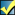A Flash Developer Resource Site

# Thread: [RESOLVED] How do you get a unique ID from two numbers w/o ordering them?

1. ##[RESOLVED] How do you get a unique ID from two numbers w/o ordering them?

Hey, not sure where else to post this...
I'm trying to group together a sql table that looks something like this:

PHP Code:
``` a_userID    |    b_userID     |    val    1            |       2              |    30    2            |       1              |    22    1            |       3              |    47  ```
... such that I can get the SUM(val) of rows where a_userID=1 and b_userID=2 and vice versa. This is at the top of a massive query that I don't want to run twice or group further, so my thought was to generate a partner key for 1+2 which would be the same key as for 2+1, but would be unique to just that combination of partners... I'm somehow tongue-tied trying to express this more clearly...

There must be some simple equation that can generate a unique number for any two number combinations ............right? Or is that a completely daft question? For some reason this has me stumped.Reply With Quote

2. hey there. do you have any example of "simple equation" to compute unique number from two, even without f(x,y)=f(y,x) condition? I mean, you can fill your tables all you want as long as numbers' number is limited, but could it really be done for ALL the numbers?

I mean, if this kind of equation exists, it must be very weird, because every z = const plane would have to intersect z = f(x,y) in just one point (not a curve).Reply With Quote

3. I think... if I didn't need f(x,y) == f(y,x), and assuming only positive integers where x != y, I could generate a unique number for each pair by doing (x*y)+y ...which gives a hyperbolic paraboloid.
However, that is dependent on the order f(x,y) and doesn't help my situation because I need a key by which to group both ways.

I've gone ahead and solved my problem by using CONCAT(MIN(userID),',',MAX(userID)) to generate a unique identifier, but unfortunately this is rather slow.Reply With Quote

4. I could generate a unique number for each pair by doing (x*y)+y
suppose you have some z = (x*y)+y. then, for some x1 != x you can find y1 = z / (x1 + 1), so that x1*y1 + y1 is the same z.Reply With Quote

5. I think this needs some special kind of space filling curve to solve this, if it's even possible at all.Reply With Quote

6. ##this gave me an idea... since I'm only looking at one quadrant, what if I took the radius of the circle needed to touch both (x,y) and (y,x)...I mean, just the hypotenuse of a right triangle touching either point... plus the sine times cosine of the vector... wouldn't that give a unique identifier?

like...

(x^2+y^2)+( SIN(ATAN(x/y)) * COS(ATAN(x/y)) )

everything right of the decimal point would be descriptive of the vector, unique because of the phase difference and because we're only dealing with one quadrant... everything left of the decimal point would be a whole integer hypotenuse^2...Reply With Quote

7. [edit: plotted it, and it looks like it should work...?]Reply With Quote

8. (x^2+y^2)+( SIN(ATAN(x/y)) * COS(ATAN(x/y)) )
unfortunately no, it does not depend on how much of full plane you use, as long as there's infinite amount of numbers. take a look at the graph, and especially contour plot of your function below - every curve on contour plot represents a set of (x,y) points that evaluate in the same value.Reply With Quote

9. yes... I know they're circular... but is there ever more than one pair of whole integers described? I mean, could any two integers produce the same result as any other two integers, so that
[ f(x1,y1) , f(y1,x1) ] == [ f(x2,y2) , f(y2,x2) ] ?
If not... then this would satisfy to give a unique identifier of two ID's combined...Reply With Quote

10. aah, integers - I kinda thought of any real numbers. it might work with integers, indeed, maybe run 100000x100000 check loop? if you have a couple of hours to wasteReply With Quote

11. It works, at least to 1000 x 1000...and I don't see why it wouldn't work infinitely =)

It also works to produce unique identifiers for reversible combinations of more than two integers, like this:

[unique floating id from a set of three integers]
(a^2+b^2+c^2)+( sin(atan(a/b))*cos(atan(a/b))*sin(atan(a/c))*cos(atan(a/c))*sin(atan(b/c))*cos(atan(b/c)) )

Solved!Reply With Quote

12. btw,which means, SIN(ATAN(x/y)) * COS(ATAN(x/y)) = TAN (ATAN (x/y)) / (1 + TAN^2 (ATAN(x/y))) = (x/y) / (1 + (x/y)^2) = (x * y) / (x^2 + y^2), right?Reply With Quote

13. Thanks! That's nifty...
feeling pretty good about solving this one =)Reply With Quote

14. Awesome, thanks for sharing your solution!Reply With Quote

####Posting Permissions

• You may not post new threads
• You may not post replies
• You may not post attachments
• You may not edit your posts
•

 » Home » Movies » Tutorials » Submissions » Board » Links » Reviews » Feedback » Gallery » Fonts » The Lounge » Sound Loops » Sound FX » About FK » Sitemap

Click Here to Expand Forum to Full Width